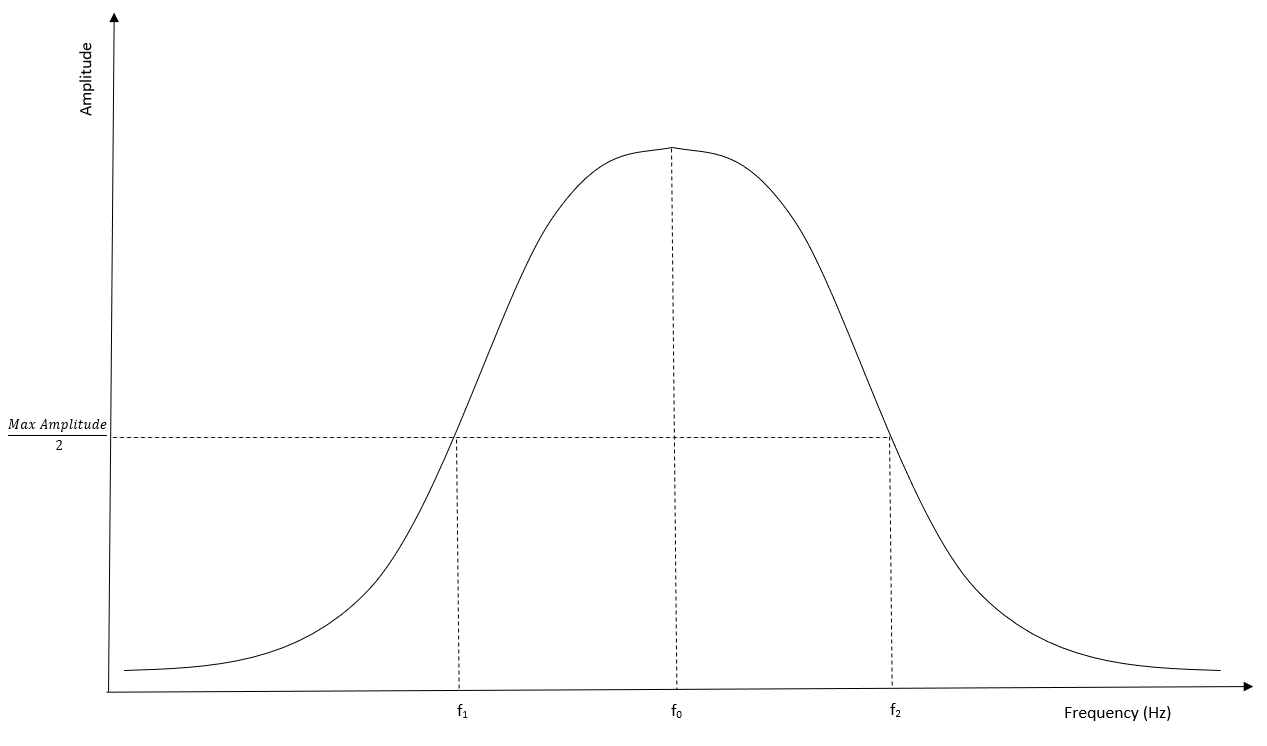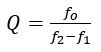# Quality Factor

Quality Factor (Q Factor) is the ratio between the center frequency fand the half conductance bandwidth. It is used to measure the damping of a resonating device.Conductance Plot

It is calculated using the following formula:Extracting Q factor requires conductance of the device. To do this, the review file (*.revinp) must contain the following code:

`pout      histname electrode vq all      /* Volt/charge time histories 1 & 2      end`

Once the input file is run, it creates a history file which is read into Review using the following code:

`symb filename = INSERT_FILENAME_HERE /* Change filename as requiredread f1 '\$filename.flxhst'           /* Read time history`

The conductance of the device can then be calculated using the following code:

`freq    file f2    wndo hann righ 1.    time pad \$npad      type real    admt f1 2 f1 3     end`

Then to get the value of f0, the #get command is used to extract the maximum peak value in the conductance curve. This value is then divided by 2 to get the values of  f & f 1 :

`symb rpeak2 = \$rpeak / 2symb #get { incros } curvcros f2 1 \$rpeak2 freq 2 \$fmin \$fmax`

This gets the frequencies at the half conductance amplitude points which can then be used along with f0  to calculate Q:

`symb Q = \$rfreq / ( \$freq2 - \$freq1 )`